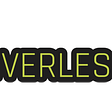# Can I use Deep Learning for that?

I often get pitched ideas around using Deep Neural Networks for some problem. Over the years the below heuristic has helped me decide whether an effort will work or whether it should be relegated to sci-fi.

# Supervised Learning

TL;DR

1. Supervised Learning is easy, you just need input data, labels and a loss function

2. Turning your problem into a Supervised Learning problem makes it easy

3. You can compose small supervised learning problems to solve hard supervised learning problems

A useful corollary is that if you can’t turn your problem into a supervised learning problem, odds are it’s really hard.

So what’s a supervised learning problem?

Given some input data x_1, x_2 … x_n

and some output data y_1, y_2 … y_n

We’d like to find a model (e.g: a neural network) f

such that we minimize the difference between our prediction f(x_i) and the correct label y_i.

If you’ve never seen the terminology above it may seem abstract so let’s visualize it.

Keep in mind that if you’re only trying to understand what you can express with a neural network then you only need to concern yourself with the shape of the input and the shape of the output. Deep neural networks have complicated insides but they’re largely irrelevant to us, we only care about the shape of the input and the output which is why we can just talk about supervised learning.

We can then represent networks like this

And now we can talk about neural networks using a visual diagram language.

Pedestrian Detection: Given a 2d image (256x256x3 width x height x # channels), output all the pedestrians in it as bounding boxes (each bounding box needs 4 points to represent, one for each corner, each point is composed of 2 real numbers)

Translation: Given a n dimensional vector where each element is a word represented in a source language output a vector where each element is a word in a target language

Spam Classifier: Given a vectorized input of observations of an email predict whether its spam or not. Any tree like or JSON like structure can be collapsed to a vector.

If you have enough examples of pairs of input and output, it means your problem is in principle solvable using neural networks.

Can we really treat neural networks as black boxes? What about SuperResNet and HyperHessianADAMDescent?

Not all problems that are expressible as a supervised learning problem are efficiently solvable which is why researchers and engineers spend a ton of time doing 5 kinds of tuning in deep neural networks

1. Hidden layer network architecture — how the neurons connect to each other and other special rules — dropout, convolution, recurrence etc..
2. Loss function — how you measure the distance between the label and the model output — euclidean, manhattan, max etc..
3. Data collection and augmentation — more data usually means a better model — image rotation, inversion, change opacity etc..
4. Optimization — the choice of algorithm to minimize the distance between the true label and the predicted label — stochastic gradient descent, 1st order methods, 2nd order methods etc..

All of these techniques bias neural networks towards certain kinds of desirable solutions more efficiently than random alternatives so they’re important if you want to make sure that your neural network works in practice but not so much when you’re trying to understand whether a neural network is the right tool for you.

# Turning Reinforcement Learning into a Supervised Learning problem

Reinforcement Learning is an immensely popular technique which has been shown to pretty much solve any sort of board game and video game (including robotic simulations). At first sight, Reinforcement Learning seems unrelated to Supervised Learning.

The basic setting is that you have an agent interacting with a world via actions. Once the agent makes a new action it observes a new state for the world and may receive a reward for its action. So far this doesn’t sound like a supervised learning problem at all. The goal for the agent is to maximize its total reward over all time or in the case of video games to win.

Reinforcement Learning turns into a Supervised learning problem by using the game simulator to generate its own input output data.

At every step of a simulation we generate a tuple (s, a, s’, r) where s is the original state, a the chosen action, s’ the next state, r the reward.

After some time we’ll have a large number of these tuples (s, a, s’, r) which we’ve generated without the help of an expensive human labeler. Reinforcement Learning becomes a Supervised Learning problem if you assume that your input is (s, a, s’) and your goal is to find a neural network that can predict the label r. At inference time you just search through the space of actions to find the one which maximizes your reward.

This idea also applies to Unsupervised Learning where you try to cluster a bunch of data points x_1, x_ 2 … x_n into a bunch of clusters C_1, c_2 … C_k where k is much smaller than n. While you don’t need any data to cluster a bunch of points, you can’t make sense of the clusters unless you label them. Active learning, semi supervised learning are all subfields of machine learning where the core idea is to label a subset of points s.t you get the full benefits of supervised learning but retain the label efficiency of unsupervised learning.

# Transformer

Transformers like GPT-3 have become immensely useful for all sorts of commercial applications around NLP. GPT-3 is unsupervised because it’s just fed the internet as raw text.

But the way Transformers are trained is very much like a supervised learning problem where you’re either doing

• Masked Language Modeling: Given tokens around word at position i, mask the i’th word and try to predict it
• Next Sentence Prediction: Given 2 sentences, which one comes first?

Both of these approaches don’t require human labeling but are creating labels directly from the dataset.

# Composing Supervised Learning problems

Suppose you don’t have a pair of input data and output labels.

You can still solve a problem if you break it down into smaller problems for which you do have input data and output labels or for which known pre-trained models exist.

This idea of breaking down a problem into smaller parts is key in concepts like recursion, dynamic programming and pretty much every single efficient algorithm out there.

We have a couple of ways combining networks to produce larger more useful networks:

1. Composition: which makes the output of the first network the input of the second network.

2. Product: × which combines the outputs of two networks into one

For example, in the context of self driving cars we’d like to combine data from a camera and a depth sensor, encode each and then pass them to a Reinforcement Learning algorithm so we figure out whether we should break, turn or accelerate

3. Inverse: f^{-1} which flips all the arrows of a neural network to solve a harder problem

So far in our diagrams we have omitted to say that the arrows are directed from input to output since it was clear from context. For each network we can produce its inverse network by flipping the arrows

A particularly compelling application the inverse is taking the inverse of the graphics problem.

Graphics is about going from a 3d scene to a 2d image which is the core of computer graphics.

Inverse graphics is about going from a 2d image to a 3d scene which is the core of scene photogrammetry.

There’s nothing magical about the representation of a 3d scene, it’s just a tree like structure where the leafs corresponds to all the objects that exist in the scene. You can flatten any tree into a vector.

As long as the inputs and outputs match you can compose smaller problems into larger ones and solve them. So next time you read an interesting paper from NeurIPS try to figure out how their work could fit into your broader pipeline.

Nerd digression

There are many more operations which let us create a compositional algebra of neural networks including foldl, unfold, cat and a bunch more different functions you would expect to see in Haskell or Lisp.

To me this is one of the most exciting and under-explored research areas in Machine Learning which is why I wrote a whole book chapter in my upcoming book: “The Robot Overlord Manual”

A programming language for Machine Learning based on Category Theory will be to Keras what Keras is to Tensorflow 1.0.

The study of composition is called Category Theory and the best introductory reference I’ve found is 7 Sketches in Compositionality by Fong and Spivak where the sections on databases and electric circuits will show you how to model the entire ML pipeline using Category Theory from data loading to processing.

Another excellent reference is GraphicalLinearAlgebra by Pawel Sobocinski which models Linear Algebra explicitly using Category Theory. Category Theory is initially intimidating because the mathematical formalism and terminology is dense but you don’t need it to get started since any algebraic statement in Category Theory can be rendered graphically. This book really drives that last point home.

Justin Le has written many blog posts about using type theory to model various problems in Machine Learning. His work has also made it to various real world Haskell projects which you can find on his github

I also really enjoyed this intuitive of explanation by BetterExplained that compares row vectors to functions/code and column vectors to data. Transpose is then just a way to go from one representation to the other.

Finally Chris Olah’s post does a great job at highlighting how recurrent networks fit into the functional paradigm.

# Hard (impossible?) problems

It’s also worth mentioning a couple of applications where it’s unclear whether they will be solved anytime soon or at all because it’s not obvious what the loss function should be.

1. Time series analysis where given a signal you try to predict its future value. It’s impossible to predict the future from the past in complex systems because you can’t predict black swans (extremely rare events). The entire financial industry is especially guilty of fraud here with complex looking but nonsensical risk models. Nostradamus’s need not apply.
2. Emotion/Empathy/Love/Passion/Sadness are poorly defined philosophically and mathematically. Same problem as Explanations, good luck formulating a good loss function.

## What’s next?

At this point you should be able to tell whether a new effort with an ML back-end is realistic or sci-fi.

If you found any of the above interesting, you will probably also enjoy my book. So please check it out and let me know if anything I said was useful to you. It always means a lot.

--

--

--

## More from Mark Saroufim

Robots will save us

Love podcasts or audiobooks? Learn on the go with our new app.

## Logging with Weights & Biases## Predicting California Wildfire Size with Neural Networks: Building A Machine Learning Project From…## Does a Computer Understand Me?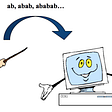## Publicis Sapient Engineering solution for Hyper-Personalization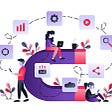## Optimizing TensorFlow Training Time for Better Performance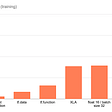## Training thousands of forecasting models at scale using Azure Automated ML and ParallelRunStep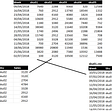## Molecular Machine Learning: Introduction to building ML models to predict molecular properties.## Logistic Regression in Brief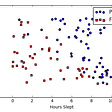## Mark Saroufim

Robots will save us

## Let’s face it: although not perfect, AI’s capabilities are pretty impressive.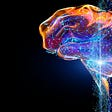## What is machine learning?## End to End Machine Learning with H2O.ai